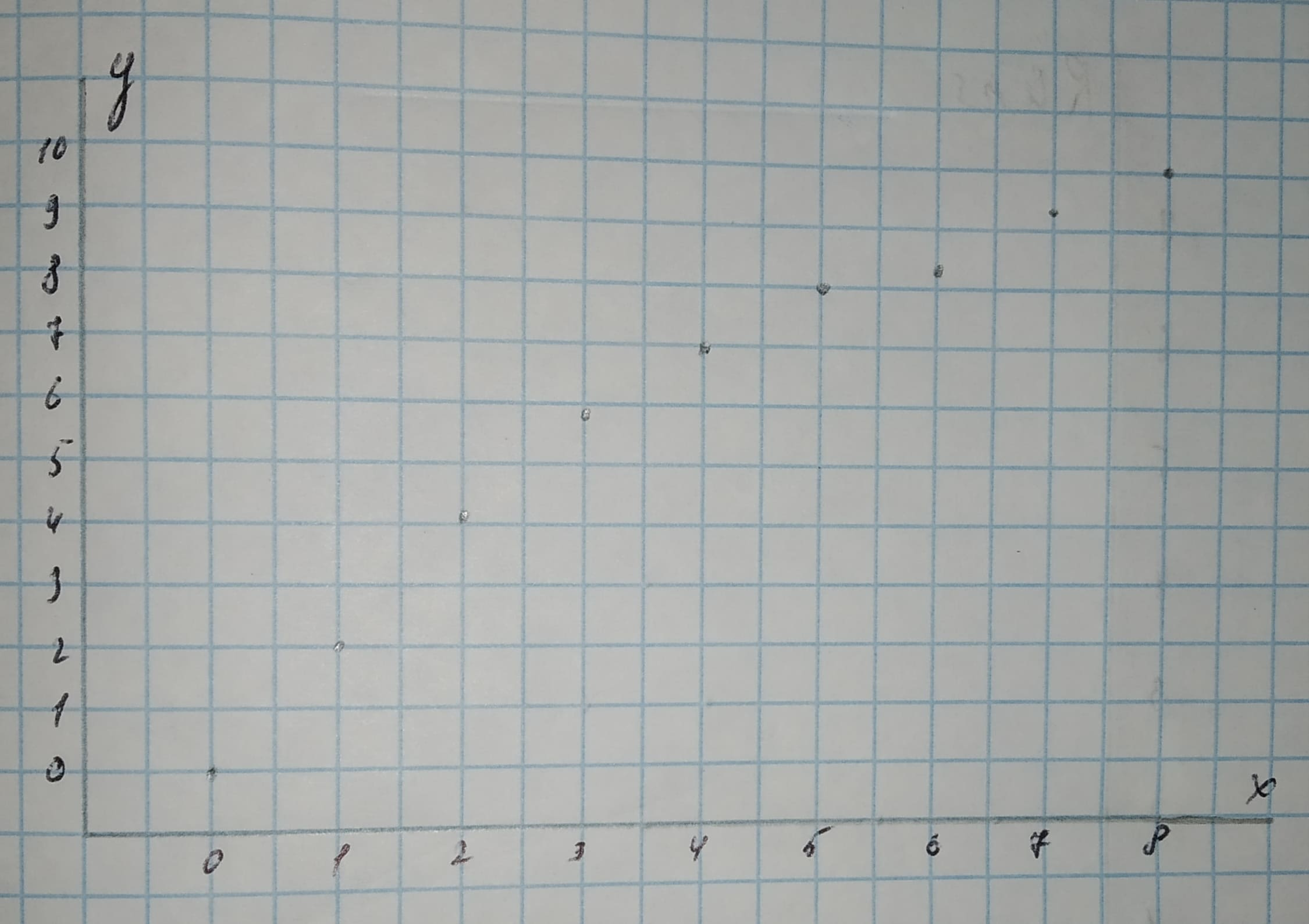Question# Make a scatterplot for each set of data. Tell whether the data show a linear association or a nonlinear association.(1,2),(7,9.5),(4,7),(2,4.2),(6,8.25),(3,5.8),(5,8),(8,10),(0,0)

Scatterplots
ANSWEREDMake a scatterplot for each set of data. Tell whether the data show a linear association or a nonlinear association.
$$(1,\ 2),\ (7,\ 9.5),\ (4,\ 7),\ (2,\ 4.2),\ (6,\ 8.25),\ (3,\ 5.8),\ (5,\ 8),\ (8,\ 10),\ (0,\ 0)$$2021-02-20

Step 1
Scatterplot
The first coordinate x in an ordered pair (x,y) is on the horizontal axis, while the second coordinate y is on the vertical axis.
The 2-values range from 0 to 8, thus an appropriate scale for the horizontal axis is from —1 to 9.
The y-values range from 0 to 10, thus an appropriate scale for the vertical axis is from —1 to 11.Step 2
We note that there is a slight curvature present in the scatterplot and thus the data show a nonlinear association.
Result:
Nonlinear assotiation.